# Lecture 6 Notebook¶

This notebook contains code that we show during lecture 6.

## MLP Model Weights¶

The purpose of this demo is to give students an intuition about what kind of weights linear models learn. We'll use the same MNIST data set that you have seen in tutorial 4 (and tutorial 6).

In :
import matplotlib.pyplot as plt
import math
import numpy as np
import torch
import torch.nn as nn
import torch.optim as optim

%matplotlib inline


Let's load the data.

In :
from torchvision import datasets, transforms

mnist_train = datasets.MNIST('data',
train=True,
transform=transforms.ToTensor())
mnist_train = list(mnist_train)[:2500]

mnist_train, mnist_val = mnist_train[:2000], mnist_train[2000:]


We'll build two models: a logistic regression model (1-layer neural network) and a 2-layer MLP:

In :
class LogisticRegression(nn.Module):
def __init__(self):
super(LogisticRegression, self).__init__()
self.layer = nn.Linear(28 * 28, 10)
def forward(self, img):
flattened = img.view(-1, 28 * 28) # flatten the image
return self.layer(flattened)

class MLP(nn.Module):
def __init__(self, num_hidden):
super(MLP, self).__init__()
self.layer1 = nn.Linear(28 * 28, num_hidden)
self.layer2 = nn.Linear(num_hidden, 10)
self.num_hidden = num_hidden
def forward(self, img):
flattened = img.view(-1, 28 * 28) # flatten the image
activation1 = self.layer1(flattened)
activation1 = torch.relu(activation1)
activation2 = self.layer2(activation1)
return activation2


Let's use the training code from tutorial 6.

In :
# code from tutorial 6
def get_accuracy(model, train=False):
if train:
data = torch.utils.data.DataLoader(mnist_train, batch_size=4096)
else:
data = torch.utils.data.DataLoader(mnist_val, batch_size=1024)

model.eval() # annotate model for evaluation
correct = 0
total = 0
for imgs, labels in data:
output = model(imgs) # We don't need to run torch.softmax
pred = output.max(1, keepdim=True) # get the index of the max log-probability
correct += pred.eq(labels.view_as(pred)).sum().item()
total += imgs.shape
return correct / total

def train(model, data, batch_size=64, weight_decay=0.0,
optimizer="sgd", learning_rate=0.1, momentum=0.9,
data_shuffle=True, num_epochs=3):
# training data
batch_size=batch_size,
shuffle=data_shuffle)
# loss function
criterion = nn.CrossEntropyLoss()
# optimizer
assert optimizer in ("sgd", "adam")
if optimizer == "sgd":
optimizer = optim.SGD(model.parameters(),
lr=learning_rate,
momentum=momentum,
weight_decay=weight_decay)
else:
lr=learning_rate,
weight_decay=weight_decay)
# track learning curve
iters, losses, train_acc, val_acc = [], [], [], []
# training
n = 0 # the number of iterations (for plotting)
for epoch in range(num_epochs):
for imgs, labels in iter(train_loader):
if imgs.size() < batch_size:
continue

model.train() # annotate model for training
out = model(imgs)
loss = criterion(out, labels)
loss.backward()
optimizer.step()

# save the current training information
iters.append(n)
losses.append(float(loss)/batch_size)             # compute *average* loss
train_acc.append(get_accuracy(model, train=True)) # compute training accuracy
val_acc.append(get_accuracy(model, train=False))  # compute validation accuracy
n += 1

# plotting
plt.title("Learning Curve")
plt.plot(iters, losses, label="Train")
plt.xlabel("Iterations")
plt.ylabel("Loss")
plt.show()

plt.title("Learning Curve")
plt.plot(iters, train_acc, label="Train")
plt.plot(iters, val_acc, label="Validation")
plt.xlabel("Iterations")
plt.ylabel("Training Accuracy")
plt.legend(loc='best')
plt.show()

print("Final Training Accuracy: {}".format(train_acc[-1]))
print("Final Validation Accuracy: {}".format(val_acc[-1]))


### Visualizing Logistic Regression weights¶

We'll visualize the weights of the logistic regression model. But first, we'll need to actually train the model.

In :
model = LogisticRegression()
train(model, mnist_train, learning_rate=0.01, optimizer="adam")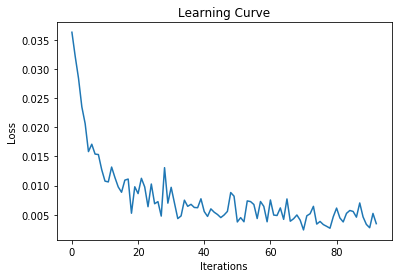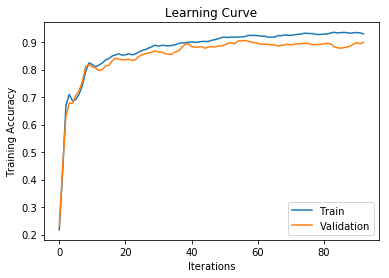Final Training Accuracy: 0.93
Final Validation Accuracy: 0.9


We can extract the weights and biases of the PyTorch model like this:

In :
W, b = list(model.layer.parameters())


Our weighst should have the shape $10 \times 784$, since there are 784 pixel inputs and 10 outputs. Each row of this matrix consists of the weights that are applied to the 784 pixels, to compute the logit associated with one of the 10 digits.

In :
W.shape

Out:
torch.Size([10, 784])

For each of the 10 digits, we'll multiply the weights that are used to compute the logit $z_k$ associated with that digit. These weights are somewhat interpretable: there is a pattern associated with each digit.

In :
W = W.reshape([10, 28, 28])

In :
weights = W.detach().numpy()

In :
plt.figure(figsize=(10, 5))
for i in range(10):
plt.subplot(2, 5, i+1)
plt.imshow(weights[i], cmap="gray")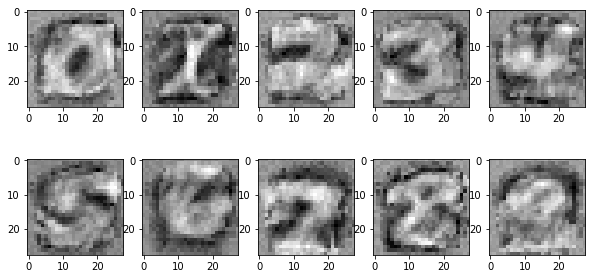### Visualizing MLP weights¶

We can visualize the weights of the first layer of a MLP in the same way. The weights of the second layer is more difficult to visualize.

In :
model = MLP(30)
train(model, mnist_train, learning_rate=0.005, optimizer="adam")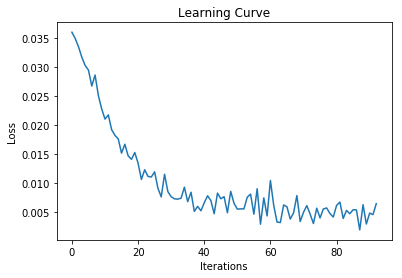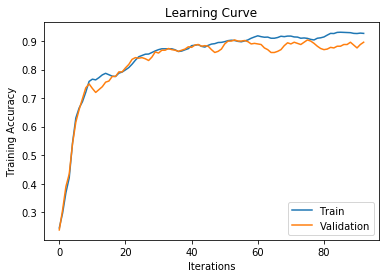Final Training Accuracy: 0.927
Final Validation Accuracy: 0.896

In :
W, b = list(model.layer1.parameters())
print(W.shape)

torch.Size([30, 784])

In :
W = W.reshape([-1, 28, 28])
weights = W.detach().numpy()
plt.figure(figsize=(10, 25))
for i in range(30):
plt.subplot(10, 5, i+1)
plt.imshow(weights[i], cmap="gray")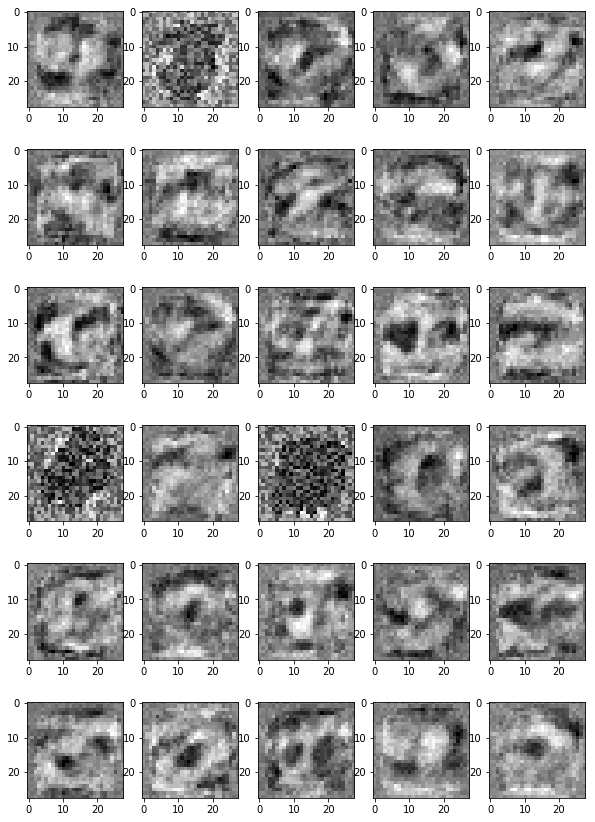One difference between these visualizations and the previous is that it is less clear what patterns these weights are trying to detect. These patterns just happens to be useful for this classification task.

# Convolutions¶

Simple convolution layer.

In :
conv = nn.Conv2d(in_channels=5, out_channels=10, kernel_size=3)
kernel, bias = list(conv.parameters())

In :
kernel.shape

Out:
torch.Size([10, 5, 3, 3])
In :
bias.shape

Out:
torch.Size()

Play around with different kernel sizes and input/output sizes.

In :
conv = nn.Conv2d(in_channels=5, out_channels=10, kernel_size=3)
kernel, bias = list(conv.parameters())
print(kernel.shape)
print(bias.shape)

torch.Size([10, 5, 3, 3])
torch.Size()


Illustrate how we can apply a convolution to an image, let's apply the (untrained) convolution to a real image. First, we load the image:

In :
img = plt.imread("imgs/dog2.jpg")
plt.imshow(img)

Out:
<matplotlib.image.AxesImage at 0x121fc00b8>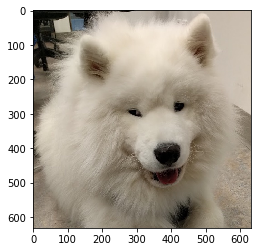PyTorch requires images to be in the "NCHW" format, meaning that image data will be a rank 4 tensor, where the dimensions are ordered in the following order:

• batching (number of elements in the batch)
• channels (the different channels in the image/feature map)
• height (of each image)
• weight (of each image)
In :
x = torch.from_numpy(img) # turn img into a PyTorch tensor
x = x.float() / 255       # turn img into a float tensor, elements between 0 and 1
print(x.shape)
x = x.permute(2,0,1)      # move the channel dimension to the beginning
print(x.shape)
x = x.reshape([1, 3, 631, 631]) # add a dimension for batching
print(x.shape)

torch.Size([631, 631, 3])
torch.Size([3, 631, 631])
torch.Size([1, 3, 631, 631])

In :
conv = nn.Conv2d(in_channels=3, out_channels=10, kernel_size=3)
y = conv(x)
y.shape

Out:
torch.Size([1, 10, 629, 629])

In :
conv = nn.Conv2d(in_channels=3, out_channels=10, kernel_size=3, padding=1)
print("Kernel:", kernel.shape)
print("Bias:", bias.shape)

y = conv(x)
print(y.shape)
print("Output units:", bias.shape)

Kernel: torch.Size([10, 5, 3, 3])
Bias: torch.Size()
torch.Size([1, 10, 631, 631])
Output units: torch.Size()


## Strided Convolution¶

In :
conv = nn.Conv2d(in_channels=3, out_channels=10, kernel_size=3, padding=1, stride=2)
print("Kernel:", kernel.shape)
print("Bias:", bias.shape)

y = conv(x)
print(y.shape)
print("Output units:", bias.shape)

Kernel: torch.Size([10, 5, 3, 3])
Bias: torch.Size()
torch.Size([1, 10, 316, 316])
Output units: torch.Size()


## Pooling¶

A pooling layer can be created like this:

In :
pool = nn.MaxPool2d(kernel_size=2, stride=2)
z = pool(y)
z.shape

Out:
torch.Size([1, 10, 158, 158])

Usually, the kernel size and the stride length will be equal.

The pooling layer has no trainable parameters:

In :
list(pool.parameters())

Out:
[]

# CNN from Tutorial 6¶

In tutorial 6, we create this CNN. We can understand this network now!

In :
class CNN(nn.Module):
def __init__(self):
super(CNN, self).__init__()
self.conv1 = nn.Conv2d(in_channels=1,
out_channels=4,
kernel_size=3,
self.pool = nn.MaxPool2d(2, 2)
self.conv2 = nn.Conv2d(in_channels=4,
out_channels=8,
kernel_size=3,
self.fc = nn.Linear(8 * 7 * 7, 10)
def forward(self, x):
x = self.pool(torch.relu(self.conv1(x)))
x = self.pool(torch.relu(self.conv2(x)))
x = x.view(-1, 8 * 7 * 7)
return self.fc(x)


This network has two convolutional layers: conv1 and conv2.

• The first convolutional layer conv1 requires an input with 1 channel, outputs 4 channels, and has a kernel size of 3x3. We are adding 1 zero padding around the image.
• The second convolutional layer conv2 requires an input with 4 channels, outputs 8 channels, and has a kernel size of (again) 3x3. We are again adding 1 zero padding around the image.

In the forward function we see that the convolution operations are always followed by the usual ReLU activation function, and a pooling operation. The pooling operation used is max pooling, so each pooling operation reduces the width and height of the neurons in the layer by half.

Because we are adding zero padding, we end up with 8 * 7 * 7 hidden units after the second convolutional layer. These units are then passed to a fully-connected layer.

Notice that the number of channels grew in later convolutional layers! However, the number of hidden units in each layer is still reduced because of the pooling operation:

• Initial Image Size: $1 \times 28 \times 28 = 784$
• After conv1: $4 \times 28 \times 28$
• After Pooling: $4 \times 14 \times 14 = 784$
• After conv2: $8 \times 14 \times 14$
• After Pooling: $8 \times 7 \times 7 = 392$
• After fc: $10$

This pattern of doubling the number of channels with every pooling / strided convolution is common in modern convolutional architectures. It is used to avoid loss of too much information within a single reduction in resolution.

# AlexNet in PyTorch (Might be lec07)¶

Convolutional networks are very commonly used, meaning that there are often alternatives to training convolutional networks from scratch. In particular, researchers often release both the architecture and the weights of the networks they train.

As an example, let's look at the AlexNet model, whose trained weights are included in torchvision. AlexNet was trained to classify images into one of many categories. The AlexNet can be imported like below.

In :
import torchvision.models

alexNet = torchvision.models.alexnet(pretrained=True)
alexNet

Out:
AlexNet(
(features): Sequential(
(0): Conv2d(3, 64, kernel_size=(11, 11), stride=(4, 4), padding=(2, 2))
(1): ReLU(inplace=True)
(2): MaxPool2d(kernel_size=3, stride=2, padding=0, dilation=1, ceil_mode=False)
(3): Conv2d(64, 192, kernel_size=(5, 5), stride=(1, 1), padding=(2, 2))
(4): ReLU(inplace=True)
(5): MaxPool2d(kernel_size=3, stride=2, padding=0, dilation=1, ceil_mode=False)
(6): Conv2d(192, 384, kernel_size=(3, 3), stride=(1, 1), padding=(1, 1))
(7): ReLU(inplace=True)
(8): Conv2d(384, 256, kernel_size=(3, 3), stride=(1, 1), padding=(1, 1))
(9): ReLU(inplace=True)
(10): Conv2d(256, 256, kernel_size=(3, 3), stride=(1, 1), padding=(1, 1))
(11): ReLU(inplace=True)
(12): MaxPool2d(kernel_size=3, stride=2, padding=0, dilation=1, ceil_mode=False)
)
(classifier): Sequential(
(0): Dropout(p=0.5, inplace=False)
(1): Linear(in_features=9216, out_features=4096, bias=True)
(2): ReLU(inplace=True)
(3): Dropout(p=0.5, inplace=False)
(4): Linear(in_features=4096, out_features=4096, bias=True)
(5): ReLU(inplace=True)
(6): Linear(in_features=4096, out_features=1000, bias=True)
)
)

Notice that the AlexNet model is split into two parts. There is a component that computes "features" using convolutions.

In :
alexNet.features

Out:
Sequential(
(0): Conv2d(3, 64, kernel_size=(11, 11), stride=(4, 4), padding=(2, 2))
(1): ReLU(inplace=True)
(2): MaxPool2d(kernel_size=3, stride=2, padding=0, dilation=1, ceil_mode=False)
(3): Conv2d(64, 192, kernel_size=(5, 5), stride=(1, 1), padding=(2, 2))
(4): ReLU(inplace=True)
(5): MaxPool2d(kernel_size=3, stride=2, padding=0, dilation=1, ceil_mode=False)
(6): Conv2d(192, 384, kernel_size=(3, 3), stride=(1, 1), padding=(1, 1))
(7): ReLU(inplace=True)
(8): Conv2d(384, 256, kernel_size=(3, 3), stride=(1, 1), padding=(1, 1))
(9): ReLU(inplace=True)
(10): Conv2d(256, 256, kernel_size=(3, 3), stride=(1, 1), padding=(1, 1))
(11): ReLU(inplace=True)
(12): MaxPool2d(kernel_size=3, stride=2, padding=0, dilation=1, ceil_mode=False)
)

There is also a component that classifies the image based on the computed features.

In :
alexNet.classifier

Out:
Sequential(
(0): Dropout(p=0.5, inplace=False)
(1): Linear(in_features=9216, out_features=4096, bias=True)
(2): ReLU(inplace=True)
(3): Dropout(p=0.5, inplace=False)
(4): Linear(in_features=4096, out_features=4096, bias=True)
(5): ReLU(inplace=True)
(6): Linear(in_features=4096, out_features=1000, bias=True)
)

## AlexNet Features¶

The first network can be used independently of the second. Specifically, it can be used to compute a set of features that can be used later on. This idea of using neural network activation features to represent images is an extremely important one, so it is important to understand the idea now.

If we take our image x from earlier and apply it to the alexNet.features network, we get some numbers like this:

In :
features = alexNet.features(x)
features.shape

Out:
torch.Size([1, 256, 18, 18])

The set of numbers in features is another way of representing our image x. Recall that our initial image x was also represented as a tensor, also a set of numbers representing pixel intensity. Geometrically speaking, we are using points in a high-dimensional space to represent the images. in our pixel representation, the axes in this high-dimensional space were different pixels. In our features representation, the axes are not as easily interpretable.

But we will want to work with the features representation, because this representation makes classification easier. This representation organizes images in a more "useful" and "semantic" way than pixels.

Let me be more specific: this set of features was trained on image classification. It turns out that these features can be useful for performing other image-related tasks as well! That is, if we want to perform an image classification task of our own (for example, classifying cancer biopsies, which is nothing like what AlexNet was trained to do), we might compute these AlexNet features, and then train a small model on top of those features. We replace the classifier portion of AlexNet, but keep its features portion intact.

Somehow, through being trained on one type of image classification problem, AlexNet learned something general about representing images for the purposes of other classification tasks.

## AlexNet First Convolutions¶

Since we have a trained model, we might as well visualize outputs of a trained convolution, to contrast with the untrained convolution we visualized earlier.

Here is the first convolution of AlexNet, applied to our image.

In :
alexNetConv = alexNet.features
y = alexNetConv(x)


The output is a $1 \times 64 \times 157 \times 157$ tensor.

In :
y = y.detach().numpy()
y = (y - y.min()) / (y.max() - y.min())
y.shape

Out:
(1, 64, 157, 157)

We can visualize each channel independently.

In :
plt.figure(figsize=(10,10))
for i in range(64):
plt.subplot(8, 8, i+1)
plt.imshow(y[0, i], cmap="gray")